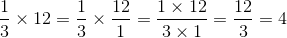ISEE Lower Level Math : Whole and Part

Example Questions

Example Question #1 : How To Find The Part From The Whole

Find the part from the whole. Round to the nearest hundredths place.

A t-shirt normally sells for $21 at the store, but today it is on sale for 25% off. What is the sale price for the t-shirt? Possible Answers: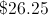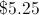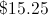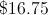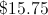Correct answer:Explanation: To find the part from the whole, multiply the "whole" by the percent (change the percent into a decimal first).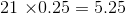The discount is$5.25.  Subtract the discount from the original price.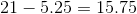.

So the t-shirt is on sale for \$15.75.

Example Question #1 : How To Find The Part From The Whole

Sara and David bake a pie and cut it into 12 equal slices. Sara eatsof the pie, and David has 1 slice before givingof the original pie to his neighbor. How many slices of pie are left?Explanation:

Sara's portion is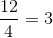While David's portion is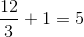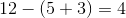Example Question #5 : How To Find The Part From The Whole

What is 43% of 82? Round to the nearest tenths place.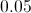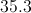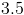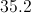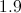Explanation:

To find the part from the whole, you will need to multiply the whole (82) by the percent. In order to do this, first change the percent to a decimal.Then, multiply the decimal and the whole.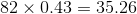43% of 82 is 35.3, rounded to the nearest tenth.

Example Question #6 : How To Find The Part From The Whole

Fred wrote down a number greater than 11 and less than 15. When Susan tried to guess it, he told her it was greater than 13 and less than 17. What is the number?

11

16

12

14

14

Explanation:

Because Fred's number is between 11 and 15, it could be 12, 13, or 14. Later he tells Susan it is greater than 13 and less than 17, so it can only be 14, since he did not change his number.

Example Question #3 : How To Find The Part From The Whole

What is 65% of 3.75?  Round to the nearest hundredths place.

2.44

2.4

2.4375

2.438

2.44

Explanation:

To find the part from the whole, you will need to multiply the whole (3.75) by the percent. In order to do this, first change the percent to a decimal (65% becomes 0.65).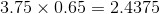Then you must round to the nearest tenths place: 2.4375 becomes 2.44.

Therefore, 65% of 3.75 is 2.44.

Example Question #121 : Isee Lower Level (Grades 5 6) Mathematics Achievement

What is four-sevenths of 490?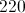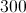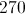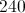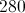Explanation:

Multiply the fraction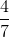by 490, putting 490 over 1 and cross-cancelling: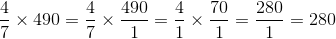Example Question #1 : How To Find The Part From The Whole

What is nine-thirteenths of 234?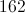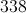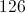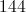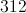Explanation:

Multiply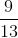by 234, rewriting and cross-cancelling: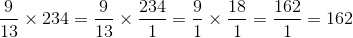Example Question #31 : Whole And Part

A pizza contains 12 slices. Becky eats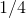of the pizza. How many slices does she eat?

6

4

5

3

3

Explanation:

Becky eatsof a pizza, which contains 12 pieces. You can find the total number that she eats by multiplying the fraction by the whole: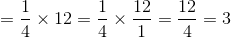Example Question #31 : Whole And Part

A total ofstudents were asked which one of three sports-- basketball, volleyball, or tennis-- they preferred. Ifstudents said basketball andsaid volleyball, how many students said they prefer tennis?Explanation:

To find out how many students prefer tennis, one should add the number of the students who prefer basketball to the number of students who prefer volleyball.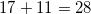The students who are not included among theseare the ones who prefer tennis. To find this number one should subtractfrom the original group ofstudents.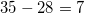Therefore,students prefer tennis.

Example Question #121 : Numbers And Operations

James ate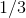of a 12-slice pizza. How many slices of pizza did he eat?

6

3

5

4